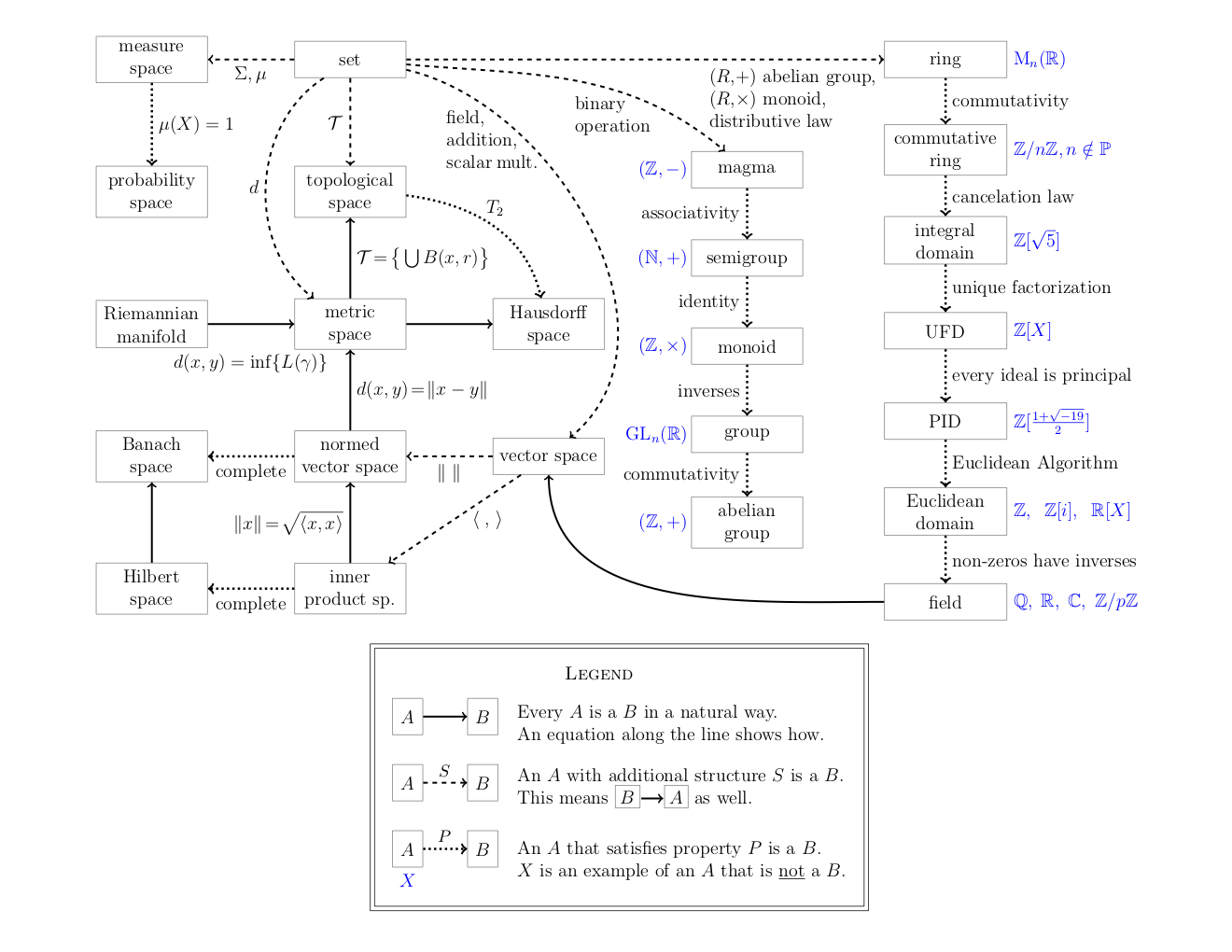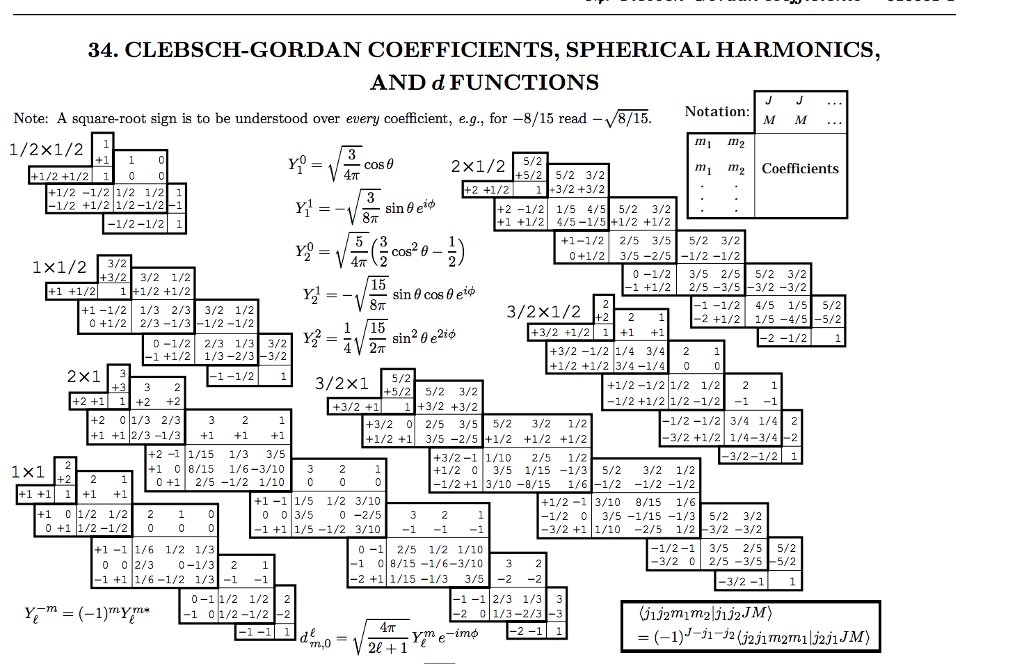# Math, Physics and Computation## Quantum mechanics## Information theory

The information is measured using the following formula

$$I(p) = \log_2\left(1\over p\right)$$

It's possible to define the Entropy for a system using an alphabet of $$q$$ simbols with probabilities $$p_i$$ as the average amount of information

$$H(P) = \sum_{i=1}^q p_i\log\left(1\over p_i\right)$$

It's possible to demonstrate that the maximum value for $$H$$ is obtained with symbols having all the same probabilities; moreover it's possible to show that

$$H(P) \leq L = \hbox{average code length}$$